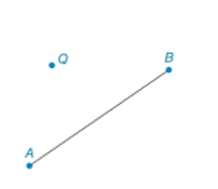Chapter 2.3, Problem 36E### Elementary Geometry for College St...

6th Edition
Daniel C. Alexander + 1 other
ISBN: 9781285195698

#### Solutions

Chapter
Section### Elementary Geometry for College St...

6th Edition
Daniel C. Alexander + 1 other
ISBN: 9781285195698
Textbook Problem
1 views

# Given that point Q does not lie on A B ¯ , construct the line through point Q that is parallel to A B ¯ .To determine

To Construct:

The parallel line.

Explanation

Given:

A line AB¯ and a point Q that does not lie on AB¯.

Figure (1)

If two lines are cut by a transversal so that a pair of corresponding angles is congruent, then these lines are parallel.

Construction:

Step (1): Consider an arbitrary point P on AB¯.

Figure (2)

Step (2): Draw a line passing through point P and point Q to become transversal EF¯

### Still sussing out bartleby?

Check out a sample textbook solution.

See a sample solution

#### The Solution to Your Study Problems

Bartleby provides explanations to thousands of textbook problems written by our experts, many with advanced degrees!

Get Started

#### Find the limit or show that it does not exist. limxx2x4+1

Single Variable Calculus: Early Transcendentals, Volume I

#### solve the equation by using the quadratic formula. 136. 3x2 4x + 1 = 0

Applied Calculus for the Managerial, Life, and Social Sciences: A Brief Approach

#### For what values of p does the series converge?

Study Guide for Stewart's Single Variable Calculus: Early Transcendentals, 8th

#### Explain the difference between a statistic and a parameter.

Research Methods for the Behavioral Sciences (MindTap Course List)